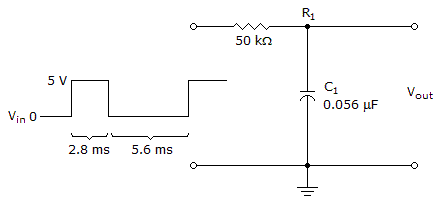# Electronics - Time Response of Reactive Circuits - Discussion

### Discussion :: Time Response of Reactive Circuits - Filling the Blanks (Q.No.1)

1.The output voltage in the given circuit is _____ at the end of the second pulse.

 [A]. 1.06 V [B]. 7.56 V [C]. 3.32 V [D]. 12 V

Explanation:

No answer description available for this question.

 Sree said: (Mar 5, 2012) Explain me please.

 Kannan said: (Jun 23, 2016) T = RC = 2.8 ms. VC = 5 * 63.3 / 100 = 3.165 v. Offtime, 2nd time constant (2*2.8 = 5.6). So, capacitor will discharge 86.5% of 3.165 v = 2.74 v. Next cycle it will again charge, Total = (3.165 - 2.74) + 5 * 63.3/100 = 0.425 + 3.165 = 3.59 v.

 Divyanshu Shekhar said: (Nov 24, 2016) RC = 50 * 10^3 * 56 * 10^-9 = 2800 * 10^-6 s =2.8 ms. For first pulse, Vc = Vo[1-e^(-τ/RC)] = 5(1-e^-2.8 ms / 2.8 ms) = 5(1-0.367) = 5 * 0.933 = 4.665 V. Between first and second pulse, Vc = [Vi * e^(-τ/RC)] = 4.665 * (e^-5.6 ms / 2.8 ms) = 4.665 * 0.135 = 0.629 V = 0.63 V(approx). For second pulse, Vc = [Vf + (Vi - Vf) * e^(-τ/RC)] = 5 + (0.63-5)e^-2.8ms /2.8ms = 5 +(-4.37) * 0.367 = 3.39 V.